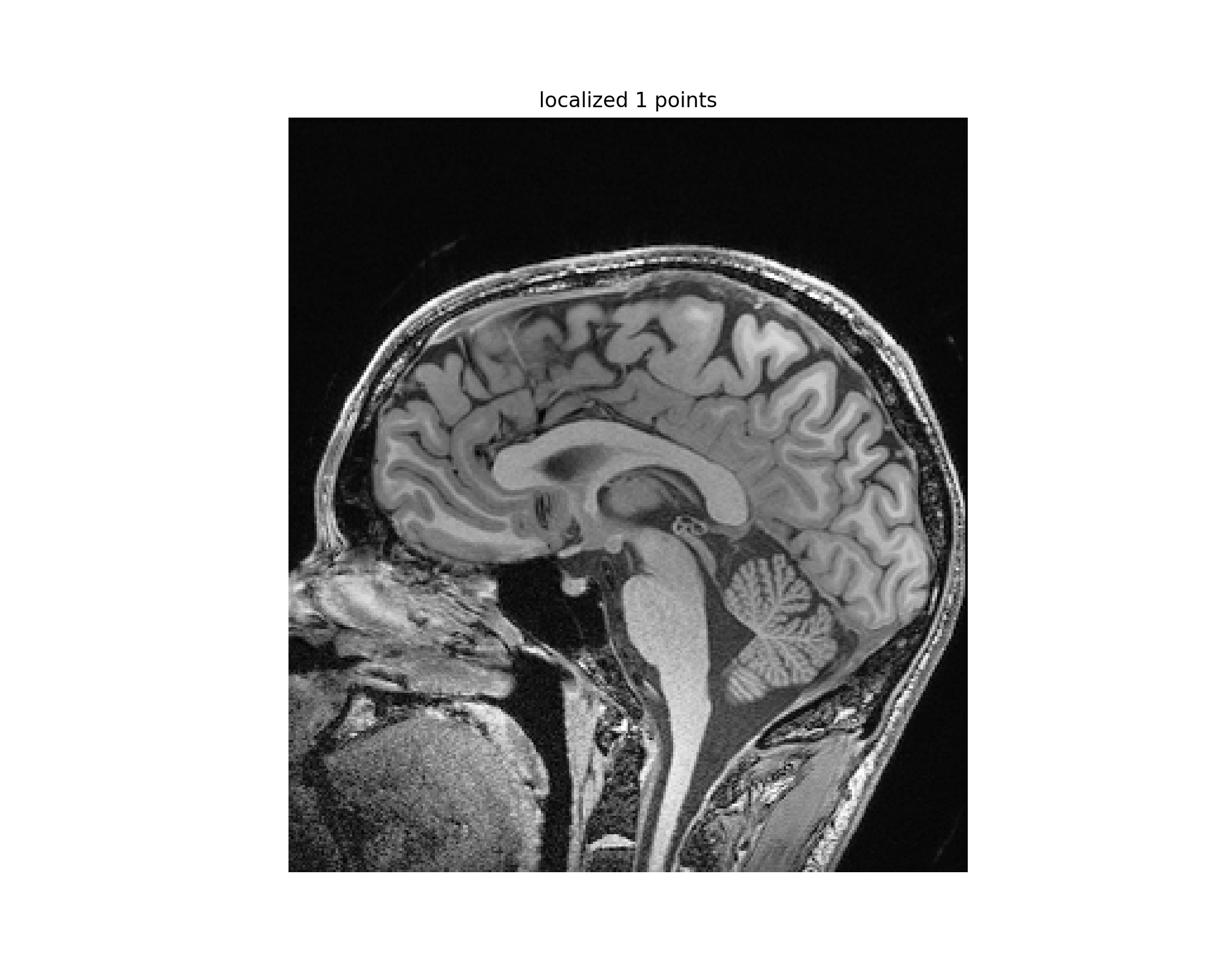# Segmentation: Region Growing

In this notebook we use one of the simplest segmentation approaches, region growing. We illustrate the use of three variants of this family of algorithms. The common theme for all algorithms is that a voxel's neighbor is considered to be in the same class if its intensities are similar to the current voxel. The definition of similar is what varies:

• ConnectedThreshold: The neighboring voxel's intensity is within explicitly specified thresholds.
• ConfidenceConnected: The neighboring voxel's intensity is within the implicitly specified bounds $\mu\pm c\sigma$, where $\mu$ is the mean intensity of the seed points, $\sigma$ their standard deviation and $c$ a user specified constant.
• VectorConfidenceConnected: A generalization of the previous approach to vector valued images, for instance multi-spectral images or multi-parametric MRI. The neighboring voxel's intensity vector is within the implicitly specified bounds using the Mahalanobis distance $\sqrt{(\mathbf{x}-\mathbf{\mu})^T\Sigma^{-1}(\mathbf{x}-\mathbf{\mu})}<c$, where $\mathbf{\mu}$ is the mean of the vectors at the seed points, $\Sigma$ is the covariance matrix and $c$ is a user specified constant.

We will illustrate the usage of these three filters using a cranial MRI scan (T1 and T2) and attempt to segment one of the ventricles.

In :
# To use interactive plots (mouse clicks, zooming, panning) we use the notebook back end. We want our graphs
# to be embedded in the notebook, inline mode, this combination is defined by the magic "%matplotlib notebook".
%matplotlib notebook

import SimpleITK as sitk

import gui

# Using an external viewer (ITK-SNAP or 3D Slicer) we identified a visually appealing window-level setting
T1_WINDOW_LEVEL = (1050,500)


## Read Data and Select Seed Point(s)¶

We first load a T1 MRI brain scan and select our seed point(s). If you are unfamiliar with the anatomy you can use the preselected seed point specified below, just uncomment the line.

In :
img_T1 = sitk.ReadImage(fdata("nac-hncma-atlas2013-Slicer4Version/Data/A1_grayT1.nrrd"))
# Rescale the intensities and map them to [0,255], these are the default values for the output
# We will use this image to display the results of segmentation
img_T1_255 = sitk.Cast(sitk.IntensityWindowing(img_T1,
windowMinimum=T1_WINDOW_LEVEL-T1_WINDOW_LEVEL/2.0,
windowMaximum=T1_WINDOW_LEVEL+T1_WINDOW_LEVEL/2.0),
sitk.sitkUInt8)

Fetching nac-hncma-atlas2013-Slicer4Version/Data/A1_grayT1.nrrd

In :
point_acquisition_interface = gui.PointDataAquisition(img_T1, window_level=(1050,500))

#preselected seed point in the left ventricle
point_acquisition_interface.set_point_indexes([(132,142,96)])In :
initial_seed_point_indexes = point_acquisition_interface.get_point_indexes()


## ConnectedThreshold¶

We start by using explicitly specified thresholds, you should modify these (lower/upper) to see the effects on the resulting segmentation.

In :
seg_explicit_thresholds = sitk.ConnectedThreshold(img_T1, seedList=initial_seed_point_indexes, lower=100, upper=170)
# Overlay the segmentation onto the T1 image
gui.MultiImageDisplay(image_list = [sitk.LabelOverlay(img_T1_255, seg_explicit_thresholds)],
title_list = ['connected threshold result'])